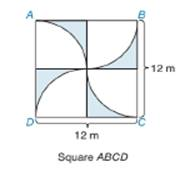Chapter 8.5, Problem 24EElementary Geometry For College St...

7th Edition
Alexander + 2 others
ISBN: 9781337614085

Solutions

Chapter
SectionElementary Geometry For College St...

7th Edition
Alexander + 2 others
ISBN: 9781337614085
Textbook Problem

In Exercises 23 and 24, find the exact areas of the shaded regions.To determine

To find:

The exact area of the shaded region.

Explanation

Formula:

Area of sector:

If r is the radius of the circle, the area A of a sector whose arc has degree measure m is given by

A=m360×πr2

Area of square:

If the length of side of a square is a, then the area of square is given by the formula:

Asquare=a2

Calculation:

To find the area of the shaded region, we have to subtract the area of 4 sectors from the area of square.

Let’s find the area of square.

The length of side of square is given as 12 m.

Asquare=a2=122=144 m2.

The horizontal and vertical segments drawn inside the square are such that they intersect the side of square at is midpoint. And hence, it is clear that the radius of each sector is equal to half of the length of side of square, that is, r=122=6 m.

Since, the horizontal segment is parallel to the sides of square, the angle formed by the horizontal segment with the side of square is 900. Thus, m=900

Let’s substitute the value of r and m to find the area of sector

Still sussing out bartleby?

Check out a sample textbook solution.

See a sample solution

The Solution to Your Study Problems

Bartleby provides explanations to thousands of textbook problems written by our experts, many with advanced degrees!

Get Started

Solve the equations in Exercises 112 for x (mentally, if possible). 2x+4=1

Finite Mathematics and Applied Calculus (MindTap Course List)

Find the general indefinite integral. sect(sect+tant)dt

Single Variable Calculus: Early Transcendentals, Volume I

In Exercises 1-6, simplify the expression. (3x)+(x+3)3

Calculus: An Applied Approach (MindTap Course List)